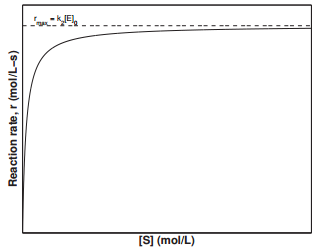# 29.9: The Michaelis-Menten Mechanism for Enzyme Catalysis

$$\newcommand{\vecs}{\overset { \rightharpoonup} {\mathbf{#1}} }$$ $$\newcommand{\vecd}{\overset{-\!-\!\rightharpoonup}{\vphantom{a}\smash {#1}}}$$$$\newcommand{\id}{\mathrm{id}}$$ $$\newcommand{\Span}{\mathrm{span}}$$ $$\newcommand{\kernel}{\mathrm{null}\,}$$ $$\newcommand{\range}{\mathrm{range}\,}$$ $$\newcommand{\RealPart}{\mathrm{Re}}$$ $$\newcommand{\ImaginaryPart}{\mathrm{Im}}$$ $$\newcommand{\Argument}{\mathrm{Arg}}$$ $$\newcommand{\norm}{\| #1 \|}$$ $$\newcommand{\inner}{\langle #1, #2 \rangle}$$ $$\newcommand{\Span}{\mathrm{span}}$$ $$\newcommand{\id}{\mathrm{id}}$$ $$\newcommand{\Span}{\mathrm{span}}$$ $$\newcommand{\kernel}{\mathrm{null}\,}$$ $$\newcommand{\range}{\mathrm{range}\,}$$ $$\newcommand{\RealPart}{\mathrm{Re}}$$ $$\newcommand{\ImaginaryPart}{\mathrm{Im}}$$ $$\newcommand{\Argument}{\mathrm{Arg}}$$ $$\newcommand{\norm}{\| #1 \|}$$ $$\newcommand{\inner}{\langle #1, #2 \rangle}$$ $$\newcommand{\Span}{\mathrm{span}}$$$$\newcommand{\AA}{\unicode[.8,0]{x212B}}$$

Enzymes are biological catalysts and functional proteins. Enzymes contain specificity in its protein structure in order to have its specialized function. It usually contains more than one subunit and they are critical to sustain life. Enzymes can increase the chemical reactions in living cells. However, enzymes are not consumed in the reaction and their main function is to assist in bringing the substrates together so they can undergo normal reaction faster.

The first enzyme was found in the process of fermentation in milk and alcohol during the nineteenth century. Later in the early 1830s, the term enzyme was used to replace the term ferment. Some scientists believe that ferments must contain living cells and some think ferments could be non-living cells. Finally, in the 1920s, Sumner purified the structure of enzyme and then properties of enzyme then was more clearly understood. Until today, enzymes are still the popular research field that many people are subject to study.

## Michaelis-Menten Kinetics

In biological systems, enzymes act as catalysts and play a critical role in accelerating reactions, anywhere from $$10^3$$ to $$10^{17}$$ times faster than the reaction would normally proceed. Enzymes are high-molecular weight proteins that act on a substrate, or reactant molecule, to form one or more products. In 1913, Leonor Michaelis and Maude Menten proposed the following reaction mechanism for enzymatic reactions:

$\text{E} + \text{S} \overset{k_1}{\underset{k_{-1}}{\rightleftharpoons}} \text{ES} \overset{k_2}{\rightarrow} \text{E} + \text{P} \nonumber$

where $$\text{E}$$ is the enzyme, $$\text{ES}$$ is the enzyme-substrate complex, and $$\text{P}$$ is the product. In the first step, the substrate binds to the active site of the enzyme. In the second step, the substrate is converted into the product and released from the substrate. For this mechanism, we can assume that the concentration of the enzyme-substrate complex, $$\text{ES}$$, is small and employ a steady-state approximation:

$\dfrac{d \left[ \text{ES} \right]}{dt} = k_1 \left[ \text{E} \right] \left[ \text{S} \right] - k_{-1} \left[ \text{ES} \right]_{ss} - k_2 \left[ \text{ES} \right]_{ss} \approx 0 \label{Eq22}$

Furthermore, because the enzyme is unchanged throughout the reaction, we express the total enzyme concentration as a sum of enzyme and enzyme-substrate complex:

$\left[ \text{E} \right]_0 = \left[ \text{ES} \right] + \left[ \text{E} \right] \label{Eq23}$

Plugging Equation $$\ref{Eq23}$$ into Equation $$\ref{Eq22}$$, we obtain

$0 = k_1 \left( \left[ \text{E} \right]_0 - \left[ \text{ES} \right]_{ss} \right) \left[ \text{S} \right] - k_{-1} \left[ \text{ES} \right]_{ss} + k_2 \left[ \text{ES} \right]_{ss} \label{Eq24}$

Solving for $$\left[ \text{ES} \right]_{ss}$$

$\left[ \text{ES} \right]_{ss} = \dfrac{k_1 \left[ \text{E} \right]_0 \left[ \text{S} \right]}{k_1 \left[ \text{S} \right] + k_{-1} + k_2} = \dfrac{\left[ \text{E} \right]_0 \left[ \text{S} \right]}{\left[ \text{S} \right] + \dfrac{k_{-1} + k_2}{k_1}} \label{Eq25}$

We can then write the reaction rate of the product as

$\dfrac{d \left[ \text{P} \right]}{dt} = k_2 \left[ \text{ES} \right]_{ss} = \dfrac{k_2 \left[ \text{E} \right]_0 \left[ \text{S} \right]}{\left[ \text{S} \right] + \dfrac{k_{-1} + k_2}{k_1}} = \dfrac{k_2 \left[ \text{E} \right]_0 \left[ \text{S} \right]}{\left[ \text{S} \right] + K_M} \label{Eq26}$

where $$K_M$$ is the Michaelis constant. Equation $$\ref{Eq26}$$ is known as the Michaelis-Menten equation. The result for Michaelis-Menten kinetics equivalent to that for a unimolecular gas phase reaction catalyzed on a solid surface. In the limit where there is a large amount of substrate present $$\left( \left[ \text{S} \right] \gg K_M \right)$$ Equation $$\ref{Eq26}$$ reduces to

$\dfrac{d \left[ \text{P} \right]}{dt} = r_\text{max} = k_2 \left[ \text{E} \right]_0 \label{Eq27}$

which is a $$0^{th}$$ order reaction, since $$\left[ \text{E} \right]_0$$ is a constant. The value $$k_2 \left[ \text{E} \right]_0$$ represents the maximum rate, $$r_\text{max}$$, at which the enzymatic reaction can proceed. The rate constant, $$k_2$$, is also known as the turnover number, which is the number of substrate molecules converted to product in a given time when all the active sites on the enzyme are occupied. Figure 29.9.4 displays the dependence of the reaction rate on the substrate concentration, $$\left[ \text{S} \right]$$. This plot is known as the Michaelis-Menten plot. Examining the figure, we can see that the reaction rate reaches a maximum value of $$k_2 \left[ \text{E} \right]_0$$ at large values of $$\left[ \text{S} \right]$$.Figure 29.9.4 : Rate dependence on substrate concentration for an enzymatic reaction.

Another commonly-used plot in examining enzyme kinetics is the Lineweaver-Burk plot, in with the inverse of the reaction rate, $$1/r$$, is plotted against the inverse of the substrate concentration $$1/\left[ \text{S} \right]$$. Rearranging Equation $$\ref{Eq26}$$,

$\dfrac{1}{r} = \dfrac{K_M + \left[ \text{S} \right]}{k_2 \left[ \text{E} \right]_0 \left[ \text{S} \right]} = \dfrac{K_M}{k_2 \left[ \text{E} \right]_0} \dfrac{1}{\left[ \text{S} \right]} + \dfrac{1}{k_2 \left[ \text{E} \right]_0} \label{Eq28}$

The Lineweaver-Burk plot results in a straight line with the slope equal to $$K_M/k_2 \left[ \text{E} \right]_0$$ and $$y$$-intercept equal to $$1/k_2 \left[ \text{E} \right]_0$$.